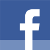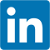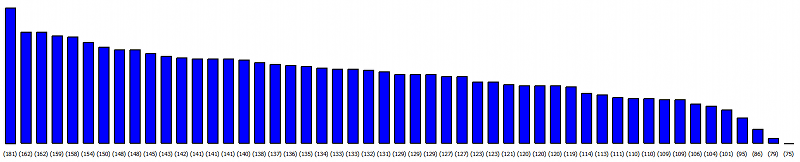# Writing a bar graph widget in Qt

published at 26.04.2014 23:20 by Jens Weller

Today I had a little fun with Qt and wrote a widget for displaying a bar graph. I have two different situations where I need the bar graph in my back end: displaying the votes a single talk got, and displaying one big bar graph of all the talks.

The display of the vote result as a bar graph brings a few more features into play, as I need a different looking bar graph in this use case. First, the labels for the bars should be the talk titles, but they are simply to long. So I need to be able to display the labels as tool tips. Then, I should also be able to cut off the bar graph, as all talks get a few votes, displaying the full height of the last bar is a little waste. The best rated talk has currently 181 voting points, the worst 75. Between them, there is a pretty even field of talks with only little difference in voting points. If I don't display the full height in the bar graph, but only the difference between best and worst, I get a much better looking graph:Currently the bar graph class is derived from QWidget:

```class BarGraph : public QWidget
{
Q_OBJECT // ugly Qt hack
std::vector<int> values; // the set of data to display
std::vector<QString> label; // the labels
int max_val,min_val,barwidth,margin = 10;
std::vector<QRect> bars; // the actual bars
bool show_tooltip=false,show_label=true,cut_min_value=false;
QBrush brush;
public:
explicit BarGraph(QWidget *parent = 0);
void paintBargraph(QPainter& painter);
//setter + getter
private://events
void paintEvent(QPaintEvent *event)override;
void mouseMoveEvent(QMouseEvent *event)override;
void resizeEvent(QResizeEvent *event)override;    void recalcBasicValues();
QString getLabel(size_t i);
};```

First to the 3 virtual functions, which enable the class to react on 3 different events:

• paint event
• mouse event
• resize event

The paint event simply creates a QPainter and forwards to paintBargraph:

```void BarGraph::paintEvent(QPaintEvent *event)
{
QPainter painter(this);
paintBargraph(painter);
}```

This is mainly to be able to later draw bar graphs in different contexts, for example into an image. The resizeEvent simply calls recalcBasicValues as the bar graph should adopt to different window sizes automatically. The mouseMoveEvent does a little more:

```void BarGraph::mouseMoveEvent(QMouseEvent *event)
{
if(!show_tooltip)
return;
QPoint pos = event->pos();
auto it = std::find_if(bars.begin(),bars.end(),[pos](const QRect& r){return r.contains(pos);});
if(it != bars.end())
{
auto i = std::distance(bars.begin(),it);
setToolTip(getLabel(i));
}
else if(!toolTip().isEmpty())
setToolTip("");
}```

It has the single duty to set the correct tooltip. This tooltip should only be displayed if the mouse is in one of the bars. QRect has a contains method which can tell me if the QPoint pos is with in this rect. With find_if and a simple lambda its now trivial to find the correct bar. But what tool tip to display if end() is not returned? As all three vectors have the same size, I can get the index of the rect with std::distance and then set the tool tip with getLabel(i). The getLabel method returns either the correct label, value or the index parameter as QString. The data to display is set via setData:

```void BarGraph::setData(std::vector val,std::vector labels)
{
values =std::move(val);
label = std::move(labels);    min_val = *std::min_element(values.begin(),values.end())-5;
if(cut_min_value)
{
for(auto& val:values)
val -= min_val;
}
max_val =* std::max_element(values.begin(),values.end());
recalcBasicValues();
setMinimumSize( (int)(margin*values.size()*2),max_val+margin*5);
}```

I haven't yet written a constructor for this as I use QtCreators UI tool. Which creates classes in with the "QObject default constructor" constructor(QObject* parent). I move the values and labels in place, as this is a sink. Then I'll need to know the min_val, and if I cut this element to 0, I'll need to substract it from every value. recalcBasicValues will now recalculate the bars:

```void BarGraph::recalcBasicValues()
{
barwidth = std::max(margin, (int)((width()-(margin*values.size()))/values.size()));

int h = height()- (margin * 4);
double factor = ((double)h) /max_val;    if(min_val < 0)
h -= min_val;
if(bars.size() != values.size())
bars.resize(values.size());
int x = margin;
for(size_t i=0, s = values.size(); i < s; ++i)
{
double barheight = values[i]*factor;
bars[i].setRect(x,h -barheight+margin,barwidth, barheight);
x += margin + barwidth;
}
}```

After resizing the bars vector (if needed), the different bars need to be (re)calculated. Each QRect is a combination of x/y and width + height. Last thing left then, is to actually draw the bar graph:

```void BarGraph::paintBargraph(QPainter &painter)
{
QPen p(Qt::black);
p.setWidth(2);
painter.setPen(p);
painter.setBrush(brush);

int y = height() - margin* 2;
QFontMetrics fm(painter.font());
int x_lbl = margin+barwidth/2;
for(size_t i=0, s = values.size(); i < s; ++i)
{
painter.drawRect(bars[i]);
if(show_label)
painter.drawText(x_lbl-fm.width(getLabel(i))/2,y,getLabel(i));
int min = cut_min_value ? min_val : 0;//if cut off, we'll need to add this value here again for correct display
QString lbl = "("+QString::number(values[i]+min)+")";
painter.drawText(x_lbl-fm.width(lbl)/2,y+fm.height(),lbl);
x_lbl += margin+barwidth;
}
}```

After the QPainter is set up, the function iterates over all values and draws the rects and label + value. With QFontMetrics the actual width of a drawn label is calculated to position it in the middle of the bar.

Of course this is only a very simple solution to draw a bar graph with a few features. Currently still missing is to label the axes. Download the code if you want to take a look at the full class.

Join the Meeting C++ patreon community!
This and other posts on Meeting C++ are enabled by my supporters on patreon!